# 120 nuts

Divide 120 nuts in a ratio of 4: 6.

a =  48
b =  72

### Step-by-step explanation:

$b=n-a=120-48=72$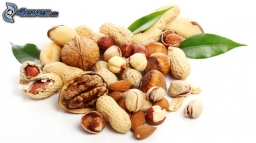Did you find an error or inaccuracy? Feel free to write us. Thank you!Tips to related online calculators
Check out our ratio calculator.
Do you want to perform natural numbers division - find the quotient and remainder?

## Related math problems and questions:

• Divide 5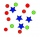Divide 288 in the following ratio 3 : 4 : 5
• The nuts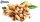There are 159 nuts in two bags, 15 more in the first than in the second, how many nuts are in the bags?
• Diofant equation250x + 120y = 5640
• Mixture of nutsThe mixture of nuts should be prepared from almonds, peanuts and cashew nuts ratio 1: 2: 3 (respectively). The price of almonds is 150 CZK/kg, the price of peanuts is 140 CZK/kg and the price of cashew nuts is 180 CZK/kg. The price of the mixture is deter
• Walnutsx walnuts were in the mission. Dano took 1/4 of nuts Michael took 1/8 from the rest and John took 34 nuts. It stayed here 29 nuts. Determine the original number of nuts.
• RemaindersIt is given a set of numbers { 170; 244; 299; 333; 351; 391; 423; 644 }. Divide this numbers by number 66 and determine set of remainders. As result write sum of this remainders.
• Nuts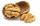How many we must have at least nuts if we can equally divide it to 10 children, 12 children or 15 children and any nut left?
• Squirrels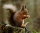The squirrels discovered a bush with hazelnuts. The first squirrel plucked one nut, the second squirrel two nuts, the third squirrel three nuts. Each new squirrel always tore one nut more than the previous squirrel. When they plucked all the nuts from the
• TogetherThe three friends divided the balls in a ratio of 6: 5: 4. Some two of them got a total of 126 balls. How many balls were there together?
• Prime divisorsFind 2/3 of the sum's ratio and the product of all prime divisors of the number 120.
• Nuts, girl and boys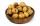Milena collected fallen nuts and called a bunch of boys let them share. She took a condition: the first boy takes one nut and tenth of the rest, the second takes 2 nuts and tenth new rest, the third takes 3 nuts and tenth new rest and so on. Thus managed
• A number 5A number is divisible by 24, 25, and 120 if it is increased by 20. Find the number.
• DivideDivide the number 72 in the ratio 7: 2 and calculate the ratio of the numbers found in this order and write down as decimal.
• Three gardensIs it possible to divide a plot of land with an area of 920 m2 into three gardens in a ratio of 4: 2: 3 so that their areas are only whole multiples of square meters?
• Collection of stampsJano, Rado, and Fero have created a collection of stamps in a ratio of 5: 6: 9. Two of them had 429 stamps together. How many stamps did their shared collection have?
• Coils of transformerWhat voltage is generated on the secondary coil of the transformer, which has 1500 turns, if the primary coil is connected to a voltage of 120 V and has 500 turns? What is the transformation ratio and what is the transformation about?
• NumbersDetermine the number of all positive integers less than 4183444 if each is divisible by 29, 7, 17. What is its sum?